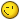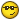#### Topic: [HACK] Restrict Users to Any Category

Name: Restrict Users to Any Category
Author: Slim
CuteNews Compatibility: 1.3.6 - 1.4.1 (higher version have a restrict userlevel to category option built in)
Description: It's something that I wanted to make long time ago, I remember asking here but the answer I got was - it's too complicated (or something like that :>).
Anyway, I've figured a way to do it, my mod gives you the option to restrict a user to any category you have while it does not depends on their access levels like the other mod, so you can restrict each user to any category.
Notes
* You can set user to one category only a.t.m but there's some ways to improve it, in the end i added a suggestion.
** The mod uses the avatar field in the user personal options so the Default Avatar URL will not work (useres even won't see it), and instead, in the avatar url in the add news page you can put whatever you want or leave it empty for the users to fill.
Instructions:
Remember to backup your files first.
Ok, here it goes:

Open options.mdu and find:

``````     if(\$member_db != 4){echo"<tr>
<td height=\"21\" width=\"200\" bgcolor=#F7F6F4>
Default Avatar URL
<td height=\"21\" width=\"400\" bgcolor=#F7F6F4 >
<input type=text name=change_avatar value=\"\$member_db\">    will appear on 'Add News' page
</tr>"; }else{ \$bg = "bgcolor=#F7F6F4"; }``````

replace with:

``````     if(\$member_db == 1){echo"<tr>
<td height=\"21\" width=\"200\" bgcolor=#F7F6F4>
Default Avatar URL
<td height=\"21\" width=\"400\" bgcolor=#F7F6F4 >
<input type=text name=change_avatar value=\"\$member_db\">    will appear on 'Add News' page
</tr>"; }else{ \$bg = "bgcolor=#F7F6F4"; }``````

This, will enable only administrators to see the default avatar input and change it in their personal options.

Save and close it, then, open addnews.mdu and find:

``````    if(\$config_use_avatar == "yes"){
echo"<tr>
<td width=\"75\">
Avatar URL
<td width=\"575\" colspan=\"2\">
<input tabindex=2 type=text size=\"42\" value=\"\$member_db\" name=\"manual_avatar\" >   <font style=\"font-size:7pt\">(optional)</font>``````

Now, you can replace the \$member_db with whatever you want to be shown at the avatar url in the add news page, if you delete it, it will be empty.

Then, find:

``````    if(count(\$cat_lines) > 0){
echo"<tr>
<td width=\"75\">
Category
<td width=\"575\" colspan=\"2\">
<select name=category tabindex=3>\n
<option value=\"\"> </option>\n";
foreach(\$cat_lines as \$single_line){
\$cat_arr = explode("|", \$single_line);
\$if_is_selected = "";
if(\$category == \$cat_arr){ \$if_is_selected = " selected "; }
echo"<option \$if_is_selected value=\"\$cat_arr\">\$cat_arr</option>\n";
}``````

And replace with:

``````    if(count(\$cat_lines) > 0){
echo"<tr>
<td width=\"75\">
Category
<td width=\"575\" colspan=\"2\">
<select name=category tabindex=3>\n
<option value=\"\"> </option>\n";
foreach(\$cat_lines as \$single_line){
\$cat_arr = explode("|", \$single_line);
if(\$member_db == \$cat_arr){
\$if_is_selected = "";
if(\$category == \$cat_arr){ \$if_is_selected = " selected "; }
echo"<option \$if_is_selected value=\"\$cat_arr\">\$cat_arr</option>\n";
}
else if(\$member_db == 1){
\$if_is_selected = "";
if(\$category == \$cat_arr){ \$if_is_selected = " selected "; }
echo"<option \$if_is_selected value=\"\$cat_arr\">\$cat_arr</option>\n";
}
}``````

This will give the users the option to choose only the category which specified in their default avatar url (I'll explain later how it will be changed).
Anyway, administrators will have all the categories, if you want editors to have them too, just change "\$member_db == 1" to "\$member_db < 3".

Save and close it.
Open editusers.mdu and find:

``````    <tr>
<td width=\"126\" height=\"20\">
<td  height=\"20\" width=\"698\">
</tr>``````

``````    <tr>
<td width=\"126\" height=\"20\">
Avatar/Cat
<td  height=\"20\" width=\"698\">
<input size=\"20\" name=\"editavatar\" value=\"\$user_arr\">
</tr>``````

This will add the avatar (which we use for the category recognition) edit option to the edit user popup (the one that pops up when you click "edit" in the Add/Edit Users page).

Then, find:

``            fwrite(\$new_db,"\$old_db_arr|\$editlevel|\$old_db_arr|\$old_db_arr|\$old_db_arr|\$old_db_arr|\$old_db_arr|\$old_db_arr|\$old_db_arr|\$old_db_arr||\n");``

And replace with:

``            fwrite(\$new_db,"\$old_db_arr|\$editlevel|\$old_db_arr|\$old_db_arr|\$old_db_arr|\$old_db_arr|\$old_db_arr|\$old_db_arr|\$editavatar|\$old_db_arr||\n");``

This will insert the new "avatar" to the database.
Save and close it, upload all the files and here you go. ^^

- So, for example, if you want a user to post only in the News category, you insert in his Avatar/Cat input - News.As I promised, here is a suggestion (mine) for enabling user/s to post on more than one category:

After you finished installing the mod open addnews.mdu and find:

``````               else if(\$member_db == 1){
\$if_is_selected = "";
if(\$category == \$cat_arr){ \$if_is_selected = " selected "; }
echo"<option \$if_is_selected value=\"\$cat_arr\">\$cat_arr</

option>\n";
}``````

``````               else if(\$member_db == \$cat_arr){
\$if_is_selected = "";
if(\$category == \$cat_arr){ \$if_is_selected = " selected "; }
echo"<option \$if_is_selected value=\"\$cat_arr\">\$cat_arr</

option>\n";
}``````

This will enable you to set for example the News and the Reviews categories's icons to "Two" and then change the wanted user's Avatar/Cat to "Two", that way he'll be able to post in those two categories.- Well, I hope I helped some of you, If you have any suggestions, additions I'll be glad to edit and improve it, I'll really appreciate your comments.
- I attached a zip file including the edited files if you want a faster way to test it.Slim